### Home > CALC > Chapter 8 > Lesson 8.1.1 > Problem8-10

8-10.
1. Use the graph of g(x) at right to answer the following questions. Provide reasons for each value found. Homework Help ✎

1.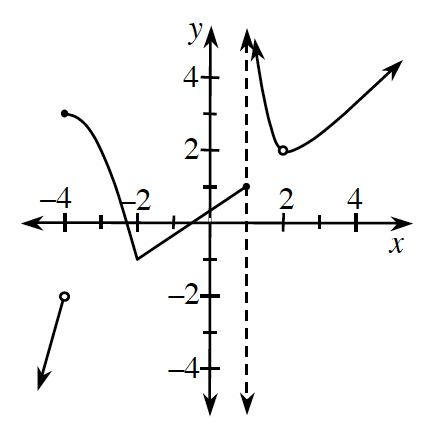For what values of x is g(x) undefined?

2. For what values of x is g(x) discontinuous?

3. For what values of x is g(x) non-differentiable?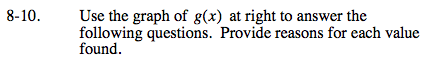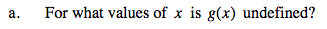Are there any x values for which there is no existing y-value?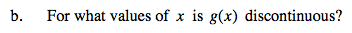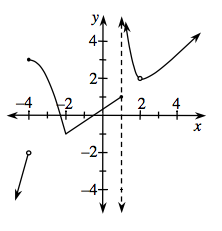There are three conditions of continuity.
If any of these conditions are violated, then the function is NOT continuous at the value of x.

$1. \ \ \lim_{x\rightarrow a}g(x)\text{ exists.} \ \ \ \text{This means that }\lim_{x\rightarrow a^{-}}g(x)=\lim_{x\rightarrow a^{+}}g(x) \text{ and that limit is finite.}$

2. f(a) exists. This means that f(a) is finite.

$3. \ \ \ \lim_{x\rightarrow a}g(x)=f(a)$

y = g(x) has exactly three points in which it is discontinuous. Where are they?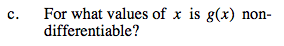A function is NOT differentiable where it is not continuous and/or where the derivative is not continuous. Examples of points of non-differentiablity include: cusps, endpoints, jumps, holes and vertical tangents.